Ex 3.4

Chapter 3 Class 10 Pair of Linear Equations in Two Variables (Term 1)
Serial order wise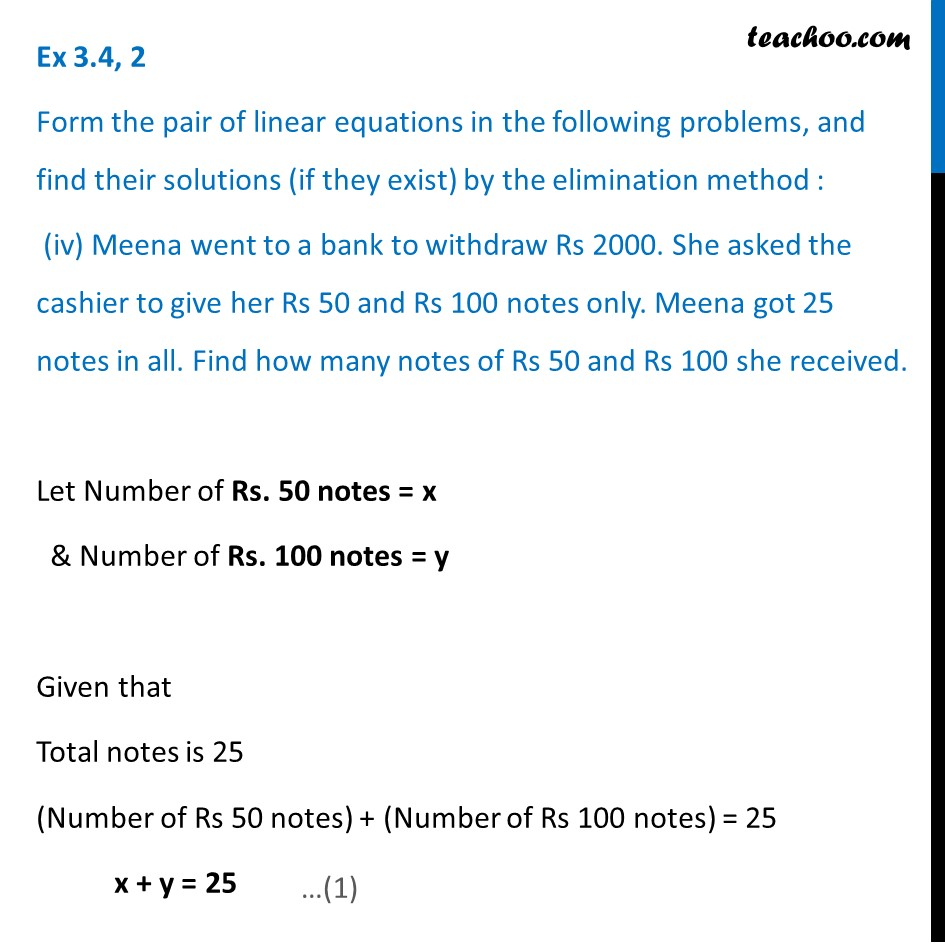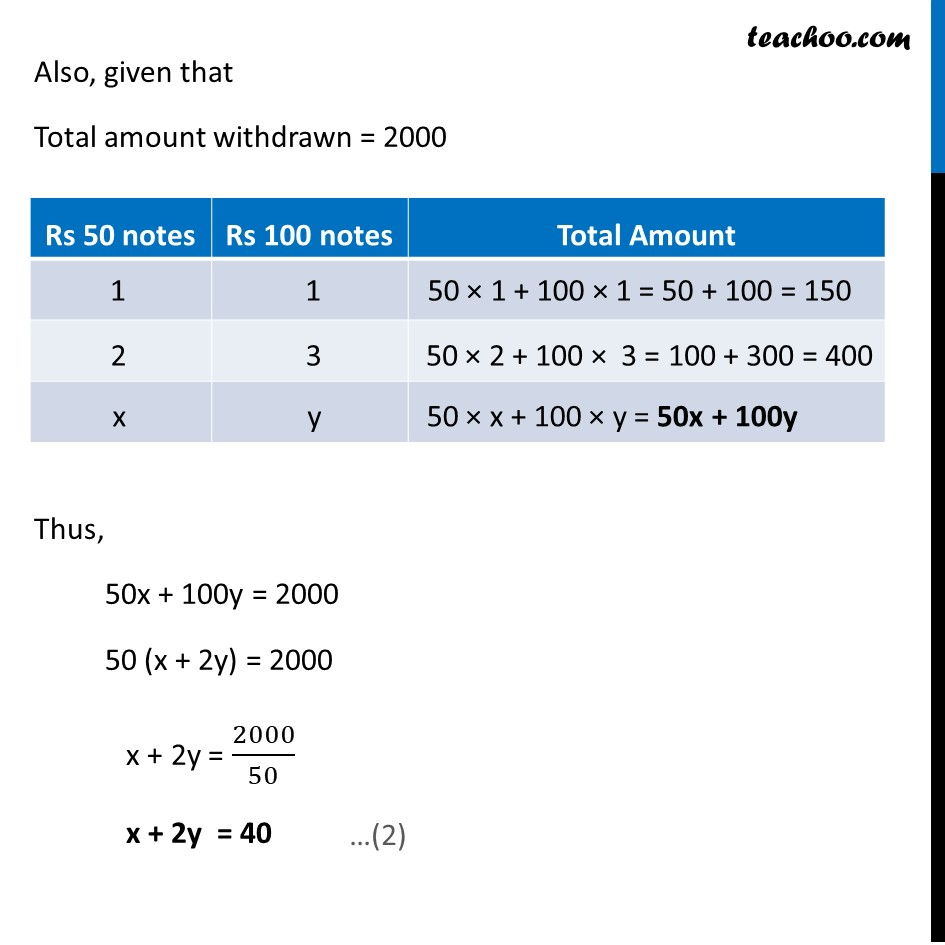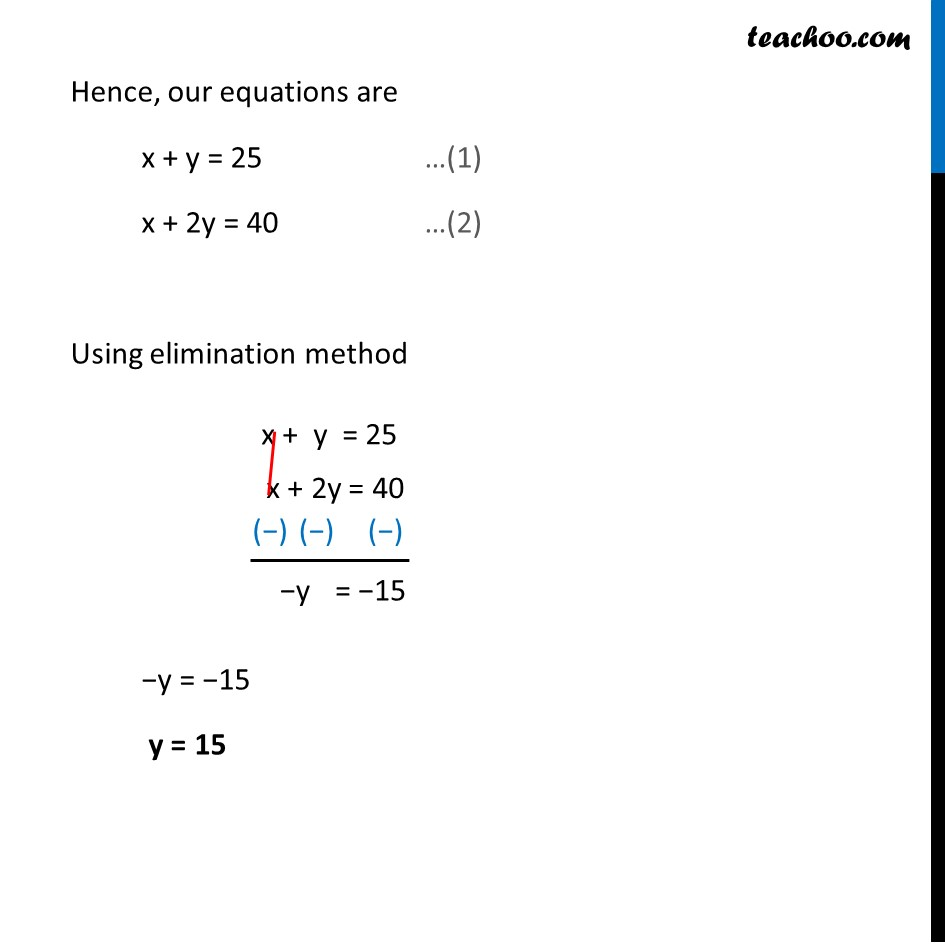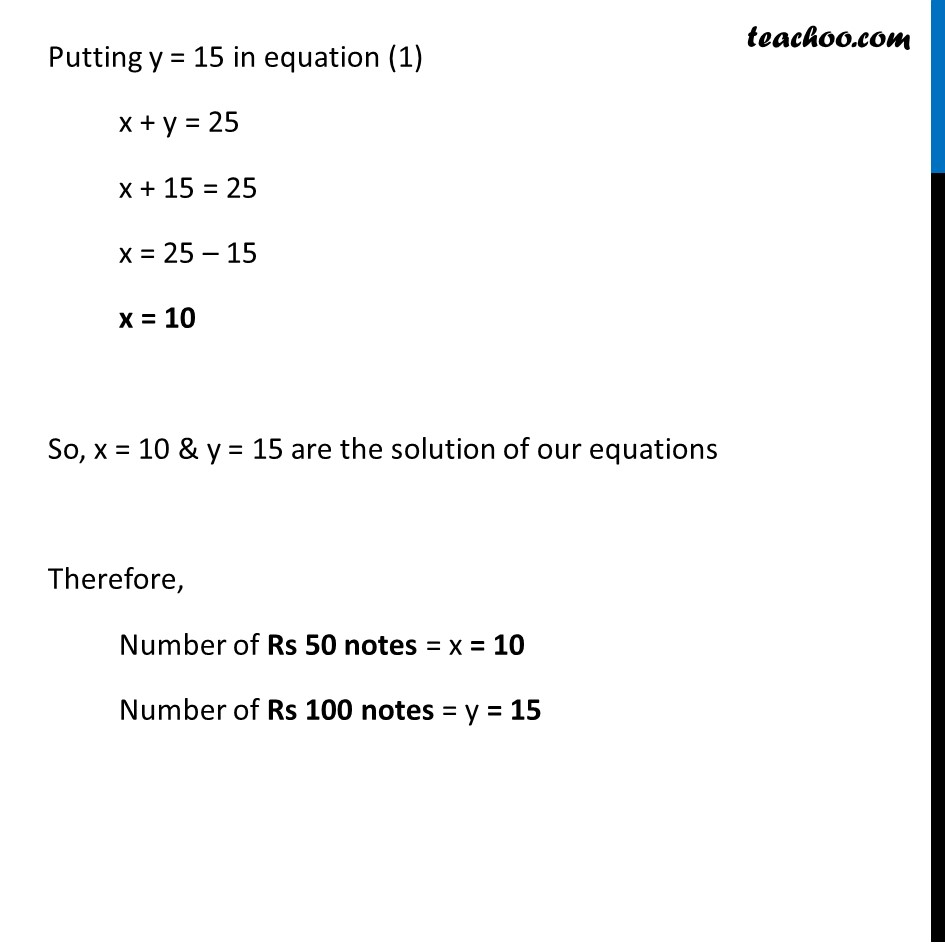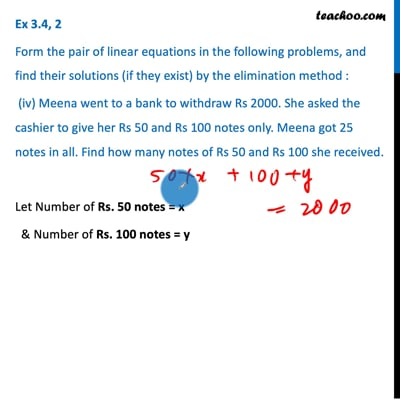This video is only available for Teachoo black users

### Transcript

Ex 3.4, 2 Form the pair of linear equations in the following problems, and find their solutions (if they exist) by the elimination method : (iv) Meena went to a bank to withdraw Rs 2000. She asked the cashier to give her Rs 50 and Rs 100 notes only. Meena got 25 notes in all. Find how many notes of Rs 50 and Rs 100 she received. Let Number of Rs. 50 notes = x & Number of Rs. 100 notes = y Given that Total notes is 25 (Number of Rs 50 notes) + (Number of Rs 100 notes) = 25 x + y = 25 Also, given that Total amount withdrawn = 2000 Thus, 50x + 100y = 2000 50 (x + 2y) = 2000 x + 2y = 2000/50 x + 2y = 40 Hence, our equations are x + y = 25 …(1) x + 2y = 40 …(2) Using elimination method −y = −15 y = 15 Putting y = 15 in equation (1) x + y = 25 x + 15 = 25 x = 25 – 15 x = 10 So, x = 10 & y = 15 are the solution of our equations Therefore, Number of Rs 50 notes = x = 10 Number of Rs 100 notes = y = 15